#### Chains and trees of bodies

For chains and trees of bodies, the ideas are conceptually the same, but the algebra becomes more cumbersome. Recall that the transformation for each link is obtained by a product of homogeneous transformation matrices, as given in (3.53) and (3.57) for the 2D and 3D cases, respectively. If the rotation part is parameterized using complex numbers for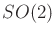or quaternions for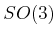, then each matrix consists of polynomial entries. After the matrix product is formed, polynomial expressions in terms of the configuration variables are obtained. Therefore, a semi-algebraic model can be constructed. For each link, all of the contact types need to be considered. Extrapolating from Examples 4.15 and 4.16, you can imagine that no human would ever want to do all of that by hand, but it can at least be automated. The ability to construct this representation automatically is also very important for the existence of theoretical algorithms that solve the motion planning problem combinatorially; see Section 6.4.

If the kinematic chains were formulated for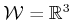using the DH parameterization, it may be inconvenient to convert to the quaternion representation. One way to avoid this is to use complex numbers to represent each of the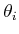and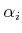variables that appear as configuration variables. This can be accomplished because only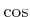and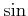functions appear in the transformation matrices. They can be replaced by the real and imaginary parts, respectively, of a complex number. The dimension will be increased, but this will be appropriately reduced after imposing the constraints that all complex numbers must have unit magnitude.

Steven M LaValle 2020-08-14